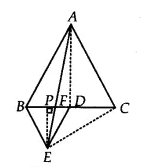Q

# In Fig.9.33, ABC and BDE are two equilateral triangles such that D is the mid-point of BC. If AE intersects BC at F, show that (iv) ar (BFE) = ar (AFD)

Q: 5        In Fig.9.33, ABC and BDE are two equilateral triangles such that D is the mid-point of BC. If AE intersects BC at F, show that

(iv)
[Hint: Join EC and AD. Show that   and  , etc.]ViewsSince ABC and  BDE are equilateral triangles.
Therefore, ACB = DBE =
BE || AC

BDE and AED are on the same base ED and between same parallels AB and DE.
Therefore, ar(BED) = ar(AED)

On subtracting EFD from both sides we get

Hence proved.

Exams
Articles
Questions